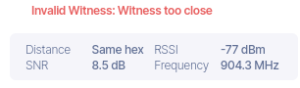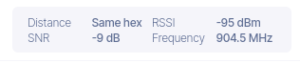# Invalid Witness – how to solve this problem

There are two types of Invalid Witness errors in Helium Mining.

### Invalid Witness: Witness RSSI too high

Every time a hotspot witnesses a beacon from another, a Helium validator will examine both received signal strength (RSSI) and the distance between the two miners.

It will then use the following information:

• the distance between the two hotspots
• antenna gains of the two hotspots

to estimate the strength of the received signals using mathematical formulas.

If the RSSI is higher than the estimate, Helium validator will declare the transaction as invalid. The witness is invalid because RSSI was higher than a reasonable threshold value. No HNT is awarded.

The reason for this is the miner transaction didn’t follow the laws of physics.

Here’s an example of such a transaction.In the above, Helium has determined that for a distance of 42 km, the RSSI level cannot be that high – it should be lower. Since it does not make sense mathematically, it’s an invalid transaction.

#### Solutions

• The location of one of the miners is incorrectly asserted. If both the transmitting miner and the receiving miner are yours, you can easily correct the location in the Helium app.
• If you’re using a high gain antenna, swap it out for one with lower gain or dBi. You could use the stock antenna that the miner shipped with

### Invalid Witness: Witness too close

If a miner witnesses another in the same Hex that’s too close (like in the same or neighboring house) the transaction is invalid. Helium determines this based on the RSSI – when it’s above a threshold. See the transaction record below.Here’s another transaction between two miners in the same Hex but unlike the one above, this one is valid.#### Solution

Move the miners further away from each other if possible.

### Summary

In this post we have described two Invalid Witness Errors and how to solve this problem.